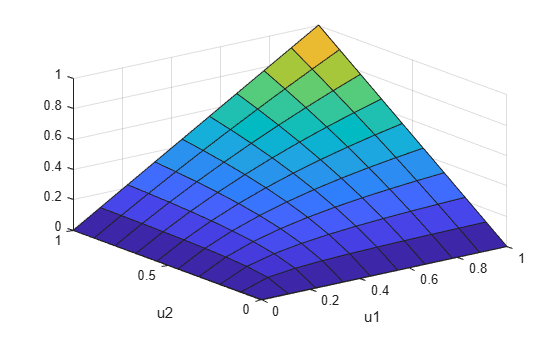# copulacdf

Copula cumulative distribution function

## Syntax

``y = copulacdf('Gaussian',u,rho)``
``y = copulacdf('t',u,rho,nu)``
``y = copulacdf(family,u,alpha)``

## Description

````y = copulacdf('Gaussian',u,rho)` returns the cumulative probability of the Gaussian copula, with linear correlation parameters `rho` evaluated at the points in `u`.```
````y = copulacdf('t',u,rho,nu)` returns the cumulative probability of the t copula, with linear correlation parameters, `rho`, and degrees of freedom parameter `nu` evaluated at the points in `u`.```

example

````y = copulacdf(family,u,alpha)` returns the cumulative probability of the bivariate Archimedean copula of the type specified by `family`, with scalar parameter `alpha` evaluated at the points in `u`.```

## Examples

collapse all

Define two 10-by-10 matrices containing the values at which to compute the cdf.

```u = linspace(0,1,10); [u1,u2] = meshgrid(u,u);```

Compute the cdf of a Clayton copula that has an alpha parameter equal to 1, at the values in `u`.

`y = copulacdf('Clayton',[u1(:),u2(:)],1);`

Plot the cdf as a surface, and label the axes.

```surf(u1,u2,reshape(y,10,10)) xlabel('u1') ylabel('u2')```## Input Arguments

collapse all

Values at which to evaluate the cdf, specified as a matrix of scalar values in the range [0,1]. If `u` is an n-by-p matrix, then its values represent n points in the p-dimensional unit hypercube. If `u` is an n-by-2 matrix, then its values represent n points in the unit square.

If you specify a bivariate Archimedean copula type (`'Clayton'`, `'Frank'`, or `'Gumbel'`), then `u` must be an n-by-2 matrix.

Data Types: `single` | `double`

Linear correlation parameters for the copula, specified as a scalar value or matrix of scalar values.

• If `u` is an n-by-p matrix, then `rho` is a p-by-p correlation matrix.

• If `u` is an n-by-2 matrix, then `rho` can be a scalar correlation coefficient.

Data Types: `single` | `double`

Degrees of freedom for the t copula, specified as a positive integer value.

Data Types: `single` | `double`

Bivariate Archimedean copula family, specified as one of the following.

 `'Clayton'` Clayton copula `'Frank'` Frank copula `'Gumbel'` Gumbel copula

Bivariate Archimedean copula parameter, specified as a scalar value. Permitted values for `alpha` depend on the specified copula family.

Copula FamilyPermitted Alpha Values
`'Clayton'`[0,∞)
`'Frank'`(-∞,∞)
`'Gumbel'`[1,∞)

Data Types: `single` | `double`

## Output Arguments

collapse all

Cumulative distribution function of the copula, evaluated at the points in `u`, returned as a vector of scalar values.

## Version History

Introduced in R2006a